﻿ Minimum uncertainty wave packet
Quantum Mechanics
Motion in a potential: Free particle

## Minimum uncertainty wave packet

A wave packet for which minimum uncertainty in both the position and the momentum is attained. In one dimension it satisfies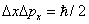, where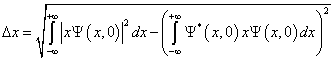is the root-mean-square deviation from the mean position and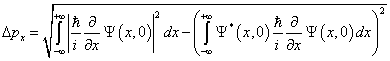is the root-mean-square from the mean momentum. The solution to the mathematical problem of finding the wave function that satisfiesis left as an exercise. The result is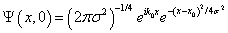where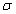is the root-mean-square deviation from the mean position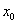and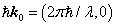is the mean momentum of the particle. The initial probability distribution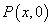is given by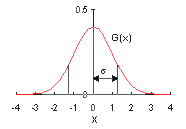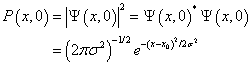a Gaussian function.is a positive real number. The Gaussian function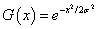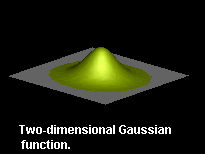is shown on the right. The curve has the same shape as the normal distribution with a standard deviation. In two or three dimensions the minimum uncertainty wave packet takes the form of a product of one-dimensional minimum uncertainty wave packets. For a particle moving in a plane the probability distribution looks like the one shown in the picture on the right.